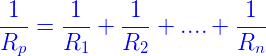# Resistors in Parallel Formula

Resistors offer resistance to the flow of current in a circuit The effective resistance depends on the connection; whether it is connected in series or parallel. In a series connection, the current will be constant whereas in parallel connection voltage will be constant through the circuit. So in household appliances, parallel connection is preferred. The formula for resistors in parallel connection is,Where R1, R2,……,Rn are the resistors connected in parallel

## Resistors in Parallel Problems

Let us discuss the solved problems of resisters in parallel connection.

### Solved Examples

Question 1: Calculate the resultant resistance of a parallel circuit containing three resistors; R1 = 2Ω, R2 = 4Ω and R3 = 6Ω?

Solution:Given value of resistors are,
R1 = 2Ω,
R2 = 4Ω,
R3 = 6Ω
The formula for resistors in parallel is,1/Rp =(1/2) +(1/4)+(1/6)

1/Rp =11/12

Rp=12/11
Rp = 1.0909Ω

Question 2: Calculate total resistance of the given parallel connection; R1 = 4Ω and R2 = 5Ω?

Solution: Given the value of resistors are,
R1 = 4Ω,
R2 = 5Ω
Formula for resistors in parallel is,
1/Rp = (1/4)+(1/5)

1/Rp = 9/20

Rp = 20/9
So, Rp = 2.222Ω

### Resistors In Series Formula

Resistors can be arranged in series form too such that the current flows through the resistors in series. Following is the table of formulas for parameters like the current, voltage and total resistance:

 The flow of current through the resistors I=I1=I2=I3…..=In The voltage drop across the resistors Vtotal=V1+V2+V3…..+Vn Total resistance equation Rtotal=R1+R2+R3…..+Rn

Stay tuned with BYJU’S to learn more on other Physics related concepts.